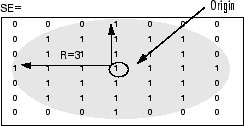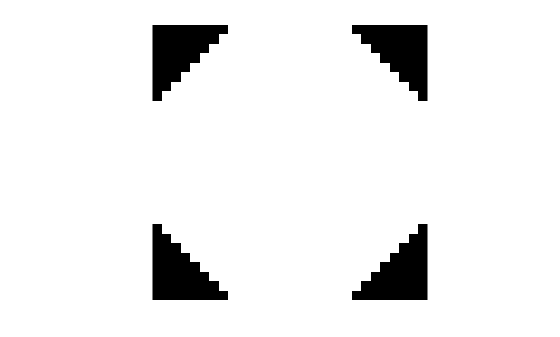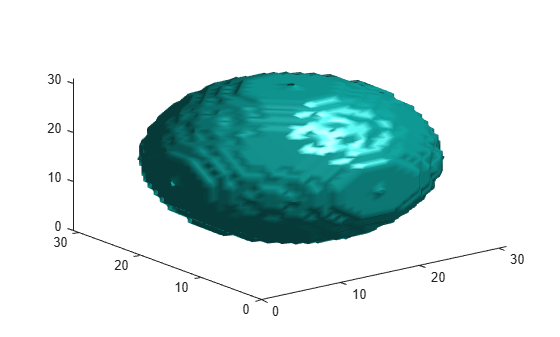strel

Morphological structuring element

Description

A strel object represents a flat morphological structuring element, which is an essential part of morphological dilation and erosion operations.

A flat structuring element is a binary valued neighborhood, either 2-D or multidimensional, in which the true pixels are included in the morphological computation, and the false pixels are not. The center pixel of the structuring element, called the origin, identifies the pixel in the image being processed. Use the strel function (described below) to create a flat structuring element. You can use flat structuring elements with both binary and grayscale images. The following figure illustrates a flat structuring element.To create a nonflat structuring element, use offsetstrel.

Creation

Description

SE = strel(nhood) creates a flat structuring element with specified neighborhood nhood.

You can also use the syntax SE = strel('arbitrary',nhood) to create a flat structuring element with a specified neighborhood.

SE = strel('diamond',r) creates a diamond-shaped structuring element, where r specifies the distance from the structuring element origin to the points of the diamond.

example

SE = strel('disk',r,n) creates a disk-shaped structuring element, where r specifies the radius and n specifies the number of line structuring elements used to approximate the disk shape. Morphological operations using disk approximations run much faster when the structuring element uses approximations.

SE = strel('octagon',r) creates a octagonal structuring element, where r specifies the distance from the structuring element origin to the sides of the octagon, as measured along the horizontal and vertical axes. r must be a nonnegative multiple of 3.

example

SE = strel('line',len,deg) creates a linear structuring element that is symmetric with respect to the neighborhood center, with approximate length len and angle deg.

SE = strel('rectangle',[m n]) creates a rectangular structuring element of size [m n].

example

SE = strel('square',w) creates a square structuring element whose width is w pixels.

SE = strel('cube',w) creates a 3-D cubic structuring element whose width is w pixels.

SE = strel('cuboid',[m n p]) creates a 3-D cuboidal structuring element of size [m n p].

example

SE = strel('sphere',r) creates a 3-D spherical structuring element whose radius is r pixels.

Compatibility

The following syntaxes still work, but offsetstrel is the preferred way to create these nonflat structuring element shapes:

• SE = strel('arbitrary',nhood,h)

• SE = strel('ball',r,h,n)

The following syntaxes still work, but are not recommended for use:

• SE = strel('pair',offset)

• SE = strel('periodicline',p,v)

Input Arguments

expand all

Neighborhood, specified as numeric array of any dimension. All nonzero pixels of nhoodbelong to the neighborhood for the morphological operation. The center (or origin) of nhood is its center element, given by floor((size(nhood) + 1)/2).

Data Types: single | double | int8 | int16 | int32 | int64 | uint8 | uint16 | uint32 | uint64 | logical

Radius of the structuring element in the x-y plane, specified as a positive integer.

• For the disk shape, r is the distance from the origin to the edge of the disk.

• For the diamond shape, r is the distance from the structuring element origin to the points of the diamond.

• For the octagon shape, r is the distance from the structuring element origin to the sides of the octagon, as measured along the horizontal and vertical axes. r must be a multiple of 3.

Data Types: double

Number of periodic line structuring elements used to approximate shape, specified as 0, 4, 6, or 8. When n is greater than 0, the disk-shaped structuring element is approximated by a sequence of n periodic-line structuring elements. When n is 0, strel does no approximation, and the structuring element members comprise all pixels whose centers are no greater than r away from the origin. Morphological operations using disk approximations run much faster when the structuring element uses approximations (n > 0). Sometimes it is necessary for strel to use two extra line structuring elements in the approximation, in which case the number of decomposed structuring elements used is n+2.

Value of nBehavior
n > 0strel uses a sequence of n (or sometimes n+2) periodic line-shaped structuring elements to approximate the shape.
n = 0strel does not use any approximation. The structuring element members comprise all pixels whose centers are no greater than r away from the origin and the corresponding height values are determined from the formula of the ellipsoid specified by r and h.

Data Types: double

Length of linear structuring element, specified as a positive number. len is approximately the distance between the centers of the structuring element members at opposite ends of the line.

Data Types: double

Angle of linear structuring element, in degrees, specified as numeric scalar. The angle is measured in a counterclockwise direction from the horizontal axis.

Data Types: double

Size of rectangular structuring element, specified as a 2-element vector of positive integers. The structuring element has m rows and n columns.

Data Types: double

Width of square or cubic structuring element, specified as a positive integer.

Data Types: double

Size of cuboidal structuring element, specified as a 3-element vector of positive integers. The structuring element has m rows, n columns, and p planes.

Data Types: double

Properties

expand all

Structuring element neighborhood, specified as a logical array.

Data Types: logical

Dimensions of structuring element, specified as a nonnegative scalar.

Data Types: double

Object Functions

 imdilate Dilate image imerode Erode image imclose Morphologically close image imopen Morphologically open image imbothat Bottom-hat filtering imtophat Top-hat filtering bwhitmiss Binary hit-miss operation
 decompose Return sequence of decomposed structuring elements reflect Reflect structuring element translate Translate structuring element

Examples

collapse all

Create an 11-by-11 square structuring element.

SE = strel('square', 11)
SE =
strel is a square shaped structuring element with properties:

Neighborhood: [11x11 logical]
Dimensionality: 2

Create a line-shaped structuring element with a length of 10 at an angle of 45 degrees.

SE = strel('line', 10, 45)
SE =
strel is a line shaped structuring element with properties:

Neighborhood: [7x7 logical]
Dimensionality: 2

View the structuring element.

SE.Neighborhood
ans = 7x7 logical array

0   0   0   0   0   0   1
0   0   0   0   0   1   0
0   0   0   0   1   0   0
0   0   0   1   0   0   0
0   0   1   0   0   0   0
0   1   0   0   0   0   0
1   0   0   0   0   0   0

Create a disk-shaped structuring element with a radius of 15.

SE3 = strel('disk', 15)
SE3 =
strel is a disk shaped structuring element with properties:

Neighborhood: [29x29 logical]
Dimensionality: 2

Display the disk-shaped structuring element.

figure
imshow(SE3.Neighborhood)Create a 3-D sphere-shaped structuring element with a radius of 15.

SE = strel('sphere', 15)
SE =
strel is a sphere shaped structuring element with properties:

Neighborhood: [31x31x31 logical]
Dimensionality: 3

Display the structuring element.

figure
isosurface(SE.Neighborhood)Tips

• Structuring elements that do not use approximations (n = 0) are not suitable for computing granulometries.

Algorithms

For all shapes except 'arbitrary', structuring elements are constructed using a family of techniques known collectively as structuring element decomposition. The principle is that dilation by some large structuring elements can be computed faster by dilation with a sequence of smaller structuring elements. For example, dilation by an 11-by-11 square structuring element can be accomplished by dilating first with a 1-by-11 structuring element and then with an 11-by-1 structuring element. This results in a theoretical performance improvement of a factor of 5.5, although in practice the actual performance improvement is somewhat less. Structuring element decompositions used for the 'disk' shape is an approximations—all other decompositions are exact.

Compatibility Considerations

expand all

Behavior changed in R2017b

 van den Boomgard, R, and R. van Balen, "Methods for Fast Morphological Image Transforms Using Bitmapped Images," Computer Vision, Graphics, and Image Processing: Graphical Models and Image Processing, Vol. 54, Number 3, pp. 252–254, May 1992.

 Adams, R., "Radial Decomposition of Discs and Spheres," Computer Vision, Graphics, and Image Processing: Graphical Models and Image Processing, Vol. 55, Number 5, pp. 325–332, September 1993.

 Jones, R., and P. Soille, "Periodic lines: Definition, cascades, and application to granulometrie," Pattern Recognition Letters, Vol. 17, pp. 1057–1063, 1996.